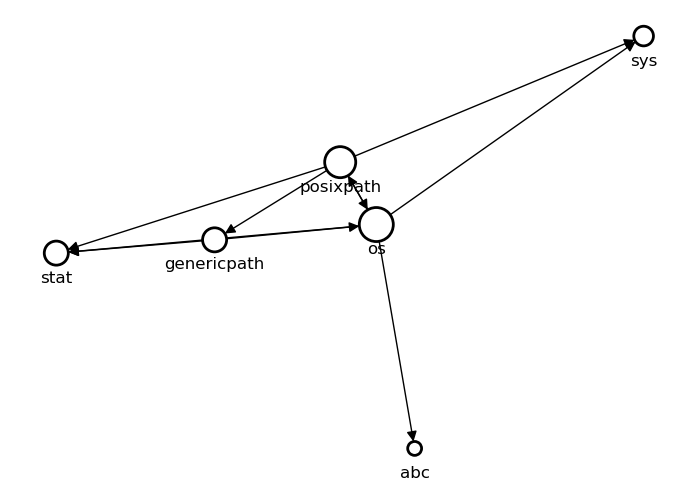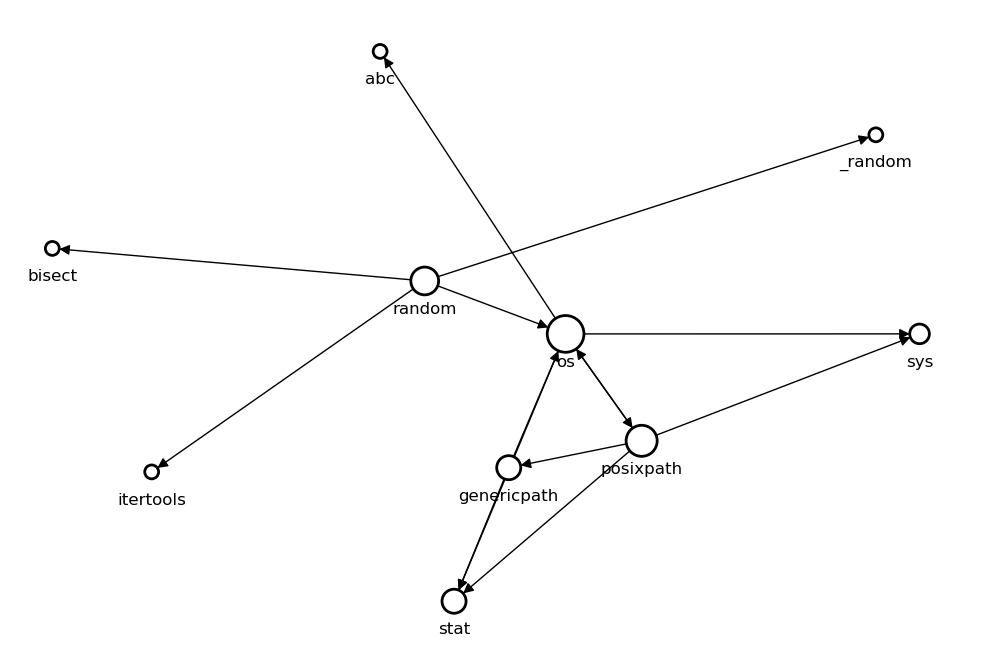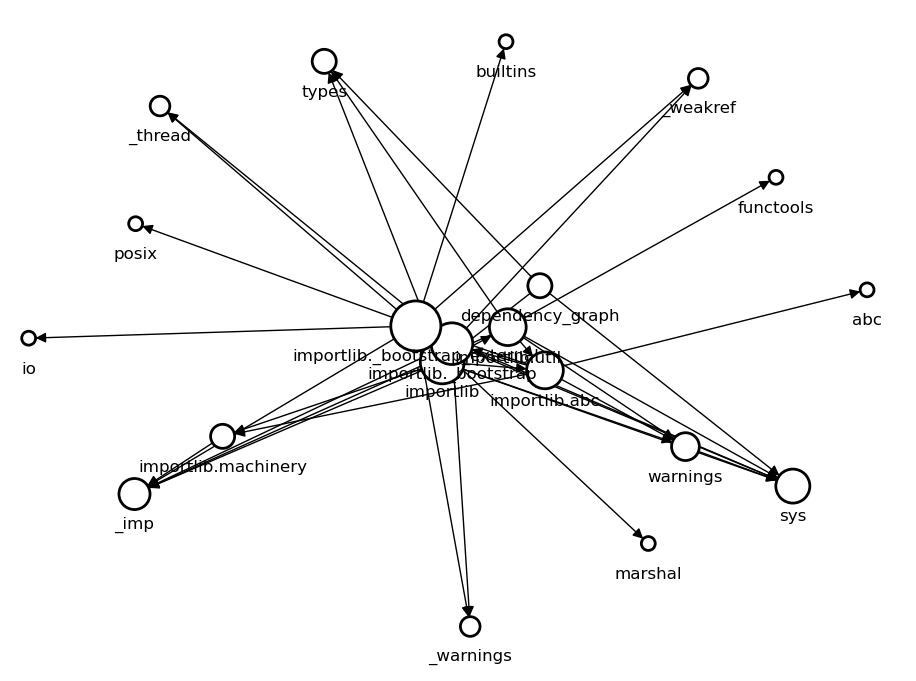## Python Module Dependency Graph

Because Python is a dynamic language, it is easy to inspect or analyze python codes on the fly. Other languages however often need source code analyses or some advanced inspecting techniques to achieve the same goal. I was reading "Learning Python" by Mark Lutz and realized imported modules are placed into symbol table `__dict__` just like other names. I thought by using `__dict__` and some type-checking it should be possible to track what modules are imported. And, we can repeat the process recursively to get the entire dependency graph. It turns out this is very easy actually and I was able to code it with less than 20 lines of code.

Of course, this method only detects modules imported with `import` statement. If a file imports a name from another module with `from <module> import <name>` statement, the method doesn't find that module. This is because `<name>` is inserted to symbol table not `<module>`. Finding those modules probably require more advanced techniques like source code or bytecode analysis. If you are looking for such a tool, look at pydeps.

The following script takes a module name as a command-line argument (Run as `python dependency_graph <module_name>`) and computes the whole dependency graph as `(V, E)` where `V` is the set of nodes and `E` is the list of edges. `importlib.import_module` is used to import a module with a given string expression because `import` statement uses the literal value of the given module name.

```import sys
import importlib
import types

def DFS(module, V, E):

module_name = module.__name__

if module_name in V:
return

for name, value in module.__dict__.items():

if(isinstance(value, types.ModuleType)):
E.append((module_name, value.__name__))
DFS(value, V, E)

if __name__ == '__main__':

module_name = sys.argv

module = importlib.import_module(module_name)

V = set()
E = list()

DFS(module, V, E)
```

We can draw this graph with networkx. The arrows represent a dependency between modules, the size of a node is scaled according to the number of dependent modules.

```import networkx as nx
import matplotlib.pyplot as plt

G = nx.DiGraph()

d = dict(nx.degree(G))

nx.draw(G, with_labels=True, nodelist=list(d.keys()),
node_size=[v * 100 for v in d.values()],
node_color='w', linewidths=2, arrowsize=15)
ax = plt.gca()
ax.collections.set_edgecolor("#000000")
plt.show()
```

### Dependency Graph of `os` Module### Dependency Graph of `random` ModuleIt can also be used for a third party or custom modules as long as those modules are in the python path so they can be importable.

### Dependency Graph of Given Script

This is the dependency graph of the script itself.